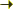Bilbao Crystallographic ServerGroup-Subgroup Correlation Relations Help

## Group-Subgroup Correlation Relations

 Group-Subgroup Correlation Relations
 The program calculates the correlation relations between the irreducible representations (irreps) of a group-subgroup pair. It takes into account all the points of the reciprocal space which are reduced to the Γ point in the low symmetry basis. Using the program one can study the behaviour of the Raman modes during phase transitions. INPUT: One have to introduce the high and low symmetry structures and the transformation matrix which relates both structures. If it is not known that matrix one can use the program STRUCTURE RELATIONS to calculate it. OUTPUT: Raman modes for the high and low symmetry structure. Modes different from Γ which might contribute in the phase transition. The correlation relations tables. NOTE: The program only accepts, as input data, structures described in a standard/default setting of the space group. If the original structure is described with respect to an ITA setting, the program SETSTRU can be used to transform it to the standard setting. In the case of more arbitrary non-conventional settings, the tool TRANSTRU can be of some help, if the transformation to the standard setting is known.

Structure Data
[in CIF format]

HINT: [ The option for a given filename is preferential ]
High Symmetry Structure
Structure Data
[in CIF format]

HINT: [ The option for a given filename is preferential ]
Low Symmetry Structure

Introduce the transformation matrix which relates both structures, you can use STRUCTURE RELATIONS to calculate this matrix. You can introduce in abc form or in matrix form.

In abc form:
In matrix form:
Linear part Origin Shift

[ Bilbao Crystallographic Server Main Menu ]